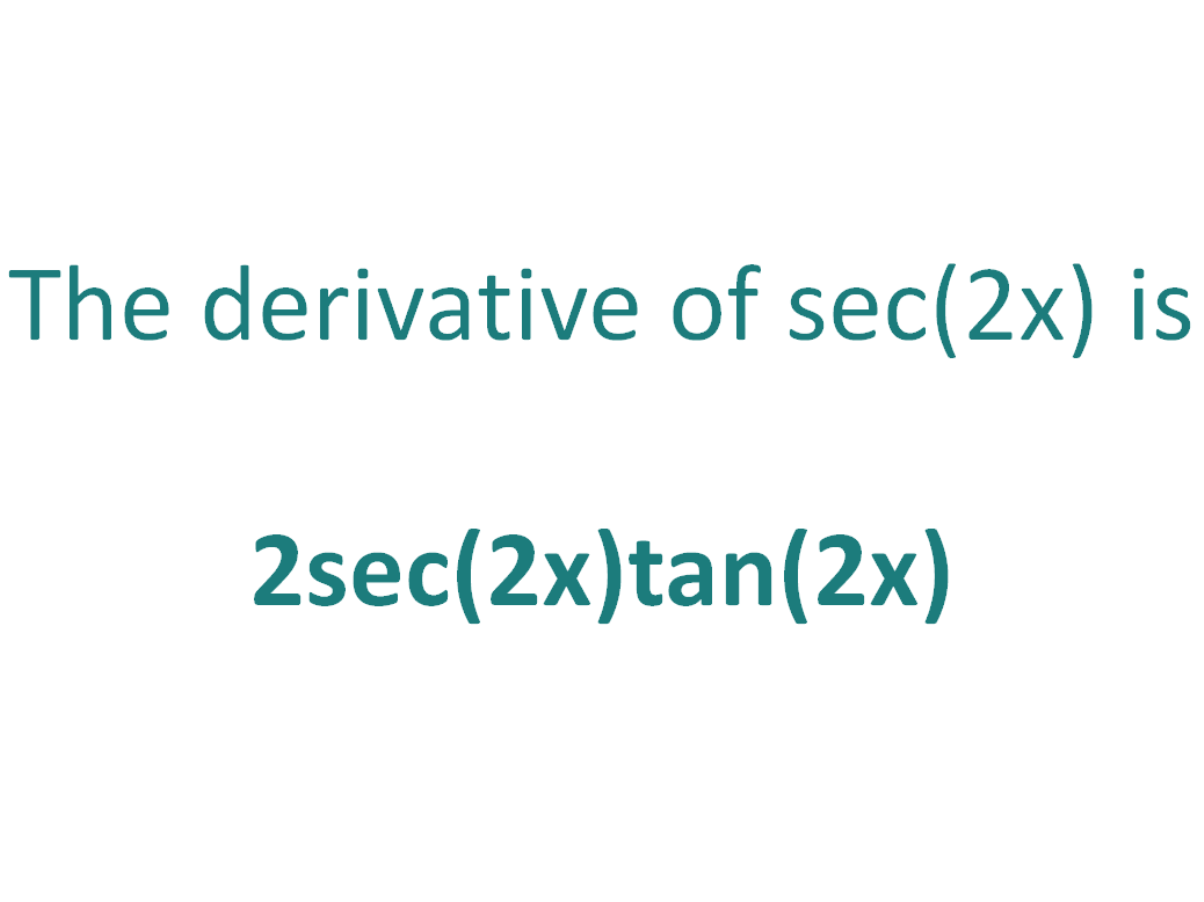# The Derivative of sec2xThe derivative of sec(2x) is 2sec(2x)tan(2x)

## How to calculate the derivative of sec(2x)

Note that in this post we will be looking at differentiating sec(2x) which is not the same as differentiating sec2(x). Here is our post dealing with how to differentiate sec^2(x).

The chain rule is useful for finding the derivative of a function which could have been differentiated had it been in x, but it is in the form of another expression which could also be differentiated if it stood on its own.

In this case:

• We know how to differentiate sec(x) (the answer is sec(x)tan(x))
• We know how to differentiate 2x (the answer is 2)

This means the chain rule will allow us to differentiate the expression sec(2x).

#### Using the chain rule to find the derivative of sec(2x)

To perform the differentiation sec(2x), the chain rule says we must differentiate the expression as if it were just in terms of x as long as we then multiply that result by the derivative of what the expression is actually in terms of (in this case the derivative of 2x).

Let’s call the function in the argument of sec, g(x), which means the function is in the form of sec(x), except it does not have x as the angle, instead it has another function of x (2x) as the angle

If:

g(x) = 2x

It follows that:

sec(2x) = sec(g(x))

So if the function f(x) = sec(x) and the function g(x) = 2x, then the function sec(2x) can be written as a composite function.

f(x) = sec(x)

f(g(x)) = sec(g(x)) (but g(x) = 2x))

f(g(x)) = sec(2x)

Let’s define this composite function as F(x):

F(x) = f(g(x)) = sec(2x)

We can find the derivative of sec(2x) (F'(x)) by making use of the chain rule.

The Chain Rule:
For two differentiable functions f(x) and g(x)

If F(x) = f(g(x))

Then the derivative of F(x) is F'(x) = f’(g(x)).g’(x)

Now we can just plug f(x) and g(x) into the chain rule. But before we do that, just a quick recap on the derivative of the sec function.

The derivative of sec(x) with respect to x is sec(x)tan(x)
The derivative of sec(z) with respect to z is sec(z)tan(z)

In a similar way, the derivative of sec(2x) with respect to 2x is sec(2x)tan(2x).

We will use this fact as part of the chain rule to find the derivative of sec(2x) with respect to x.

How to find the derivative of sec(2x) using the Chain Rule:

Using the chain rule, the derivative of sec(2x) is 2sec(2x)tan(2x)

Finally, just a note on syntax and notation: sec(2x) is sometimes written in the forms below (with the derivative as per the calculation above). Just be aware that not all of the forms below are mathematically correct.

## The Second Derivative Of sec(2x)

To calculate the second derivative of a function, you just differentiate the first derivative.

From above, we found that the first derivative of sec(2x) = 2sec(2x)tan(2x). So to find the second derivative of sec(2x), we just need to differentiate 2sec(2x)tan(2x)

We can use the chain rule to find the derivative of 2sec(2x)tan(2x) and it gives us a result of 8sec3(2x) – 4sec(2x)

The second derivative of sec(2x) is 8sec3(2x) – 4sec(2x)